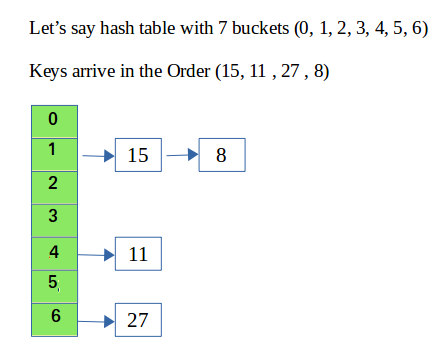# C++ program for hashing with chaining

• Difficulty Level : Medium
• Last Updated : 27 Aug, 2022

In hashing there is a hash function that maps keys to some values. But these hashing function may lead to collision that is two or more keys are mapped to same value. Chain hashing avoids collision. The idea is to make each cell of hash table point to a linked list of records that have same hash function value.

Let’s create a hash function, such that our hash table has ‘N’ number of buckets.

To insert a node into the hash table, we need to find the hash index for the given key. And it could be calculated using the hash function.

Example: hashIndex = key % noOfBuckets
Insert: Move to the bucket corresponds to the above calculated hash index and insert the new node at the end of the list.
Delete: To delete a node from hash table, calculate the hash index for the key, move to the bucket corresponds to the calculated hash index, search the list in the current bucket to find and remove the node with the given key (if found).Please refer Hashing | Set 2 (Separate Chaining) for details.
We use a list in C++ which is internally implemented as linked list (Faster insertion and deletion).

Implementation:

## CPP

 `// CPP program to implement hashing with chaining` `#include` `using` `namespace` `std;`   `class` `Hash` `{` `    ``int` `BUCKET;    ``// No. of buckets`   `    ``// Pointer to an array containing buckets` `    ``list<``int``> *table;` `public``:` `    ``Hash(``int` `V);  ``// Constructor`   `    ``// inserts a key into hash table` `    ``void` `insertItem(``int` `x);`   `    ``// deletes a key from hash table` `    ``void` `deleteItem(``int` `key);`   `    ``// hash function to map values to key` `    ``int` `hashFunction(``int` `x) {` `        ``return` `(x % BUCKET);` `    ``}`   `    ``void` `displayHash();` `};`   `Hash::Hash(``int` `b)` `{` `    ``this``->BUCKET = b;` `    ``table = ``new` `list<``int``>[BUCKET];` `}`   `void` `Hash::insertItem(``int` `key)` `{` `    ``int` `index = hashFunction(key);` `    ``table[index].push_back(key); ` `}`   `void` `Hash::deleteItem(``int` `key)` `{` `  ``// get the hash index of key` `  ``int` `index = hashFunction(key);`   `  ``// find the key in (index)th list` `  ``list <``int``> :: iterator i;` `  ``for` `(i = table[index].begin();` `           ``i != table[index].end(); i++) {` `    ``if` `(*i == key)` `      ``break``;` `  ``}`   `  ``// if key is found in hash table, remove it` `  ``if` `(i != table[index].end())` `    ``table[index].erase(i);` `}`   `// function to display hash table` `void` `Hash::displayHash() {` `  ``for` `(``int` `i = 0; i < BUCKET; i++) {` `    ``cout << i;` `    ``for` `(``auto` `x : table[i])` `      ``cout << ``" --> "` `<< x;` `    ``cout << endl;` `  ``}` `}`   `// Driver program ` `int` `main()` `{` `  ``// array that contains keys to be mapped` `  ``int` `a[] = {15, 11, 27, 8, 12};` `  ``int` `n = ``sizeof``(a)/``sizeof``(a);`   `  ``// insert the keys into the hash table` `  ``Hash h(7);   ``// 7 is count of buckets in` `               ``// hash table` `  ``for` `(``int` `i = 0; i < n; i++) ` `    ``h.insertItem(a[i]);  `   `  ``// delete 12 from hash table` `  ``h.deleteItem(12);`   `  ``// display the Hash table` `  ``h.displayHash();`   `  ``return` `0;` `}`

Output:

```0
1 --> 15 --> 8
2
3
4 --> 11
5
6 --> 27```

Time Complexity:

• Search : O(1+(n/m))
• Delete : O(1+(n/m))
where n =   Number of keys to be inserted .
m = Number of slots in Hash table .
• Here n/m is the Load Factor.
• Load Factor (∝) must be as small as possible.
• If load factor increases,then possibility of collision increases.
• Load factor is trade of space and time .
• Assume , uniform distribution of keys ,
• Expected chain length : O(∝)
• Expected time to search : O( 1 + ∝ )
• Expected time to insert/ delete :  O( 1 + ∝ )

Auxiliary Space : O(1), since no extra space has been taken.

My Personal Notes arrow_drop_up
Recommended Articles
Page :